Register for Maths, Science, English, Reasoning Olympiad Exams Register here | Book Free Demo for Live Olympiad Classes here | Check Olympiad Exam Dates here | Buy Practice Papers for IMO, IOM, HEO, IOEL etc here | Login here to participate in all India free mock test on every Saturday

# Geometrical Skills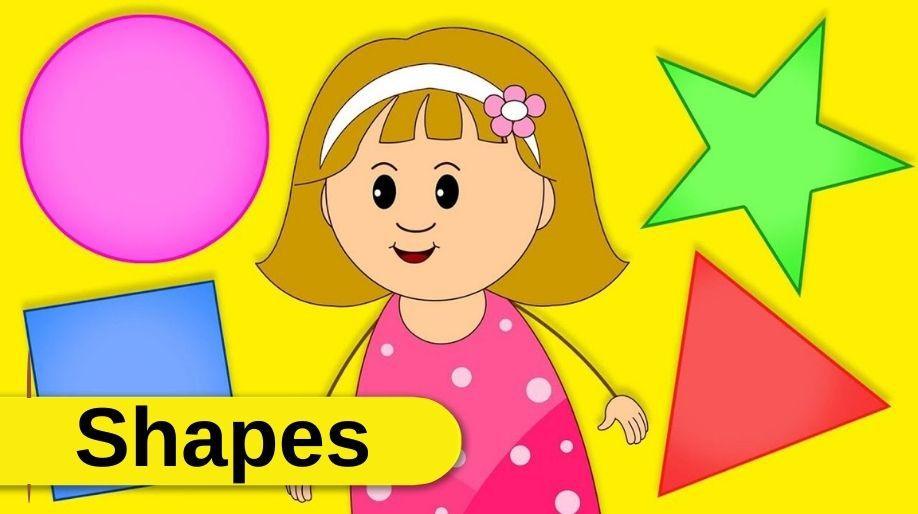Geometrical skill is ability of a person to decide about shape of any object. Have you thought was is the shape of apple that you eats daily or what is the shape of mountain? Have you thought was shape of your pencil box? Let us know about shape of all things together.

Types of geometrical Figures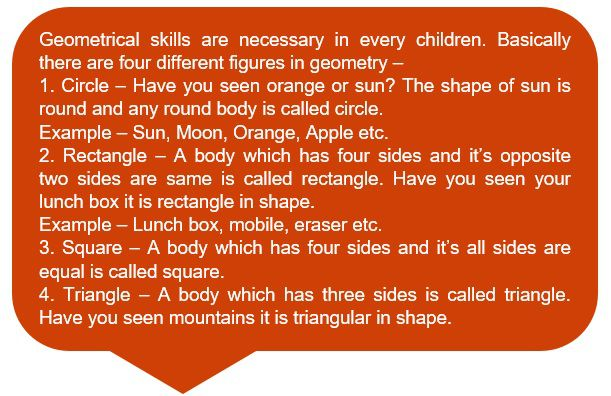Pictorial view of Geometrical figures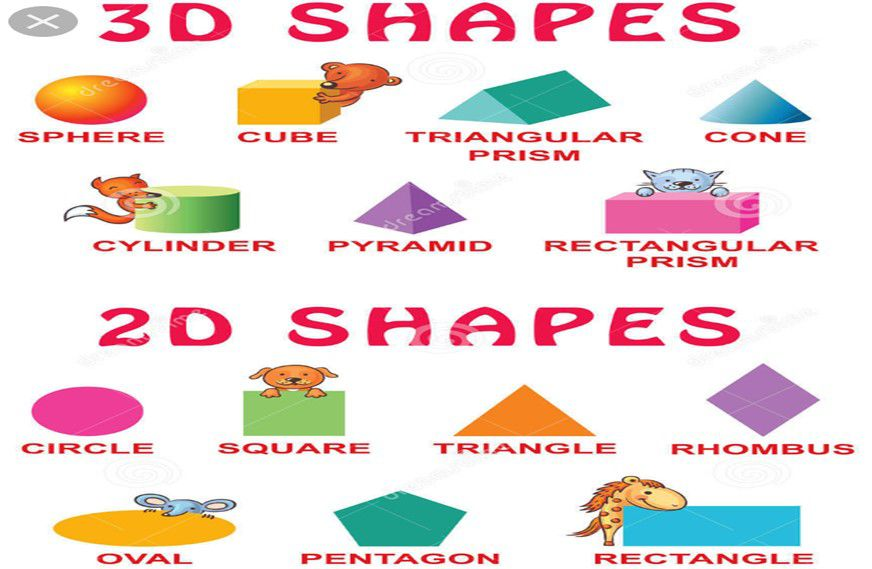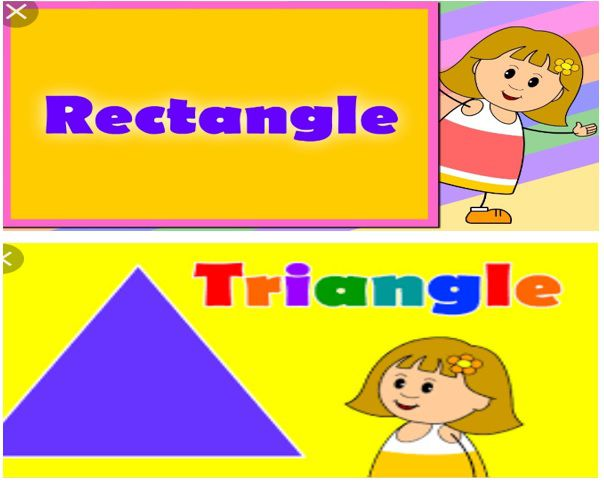Solved and unsolved examples on Geometrical skills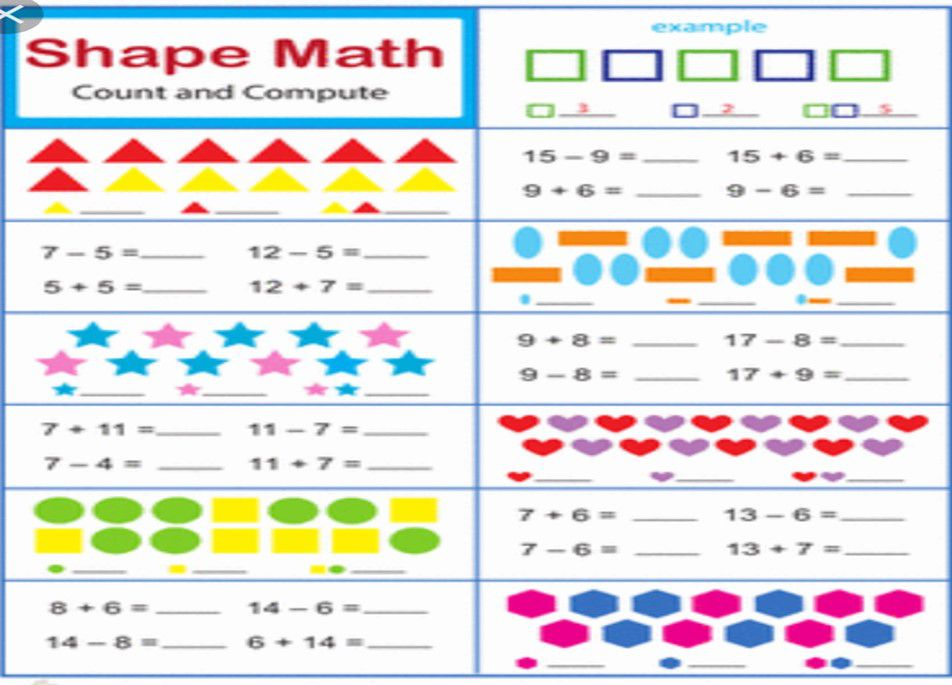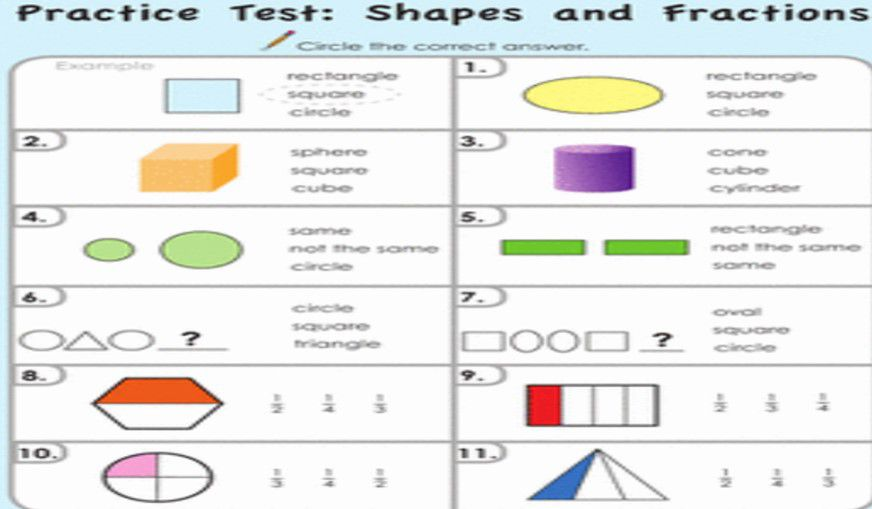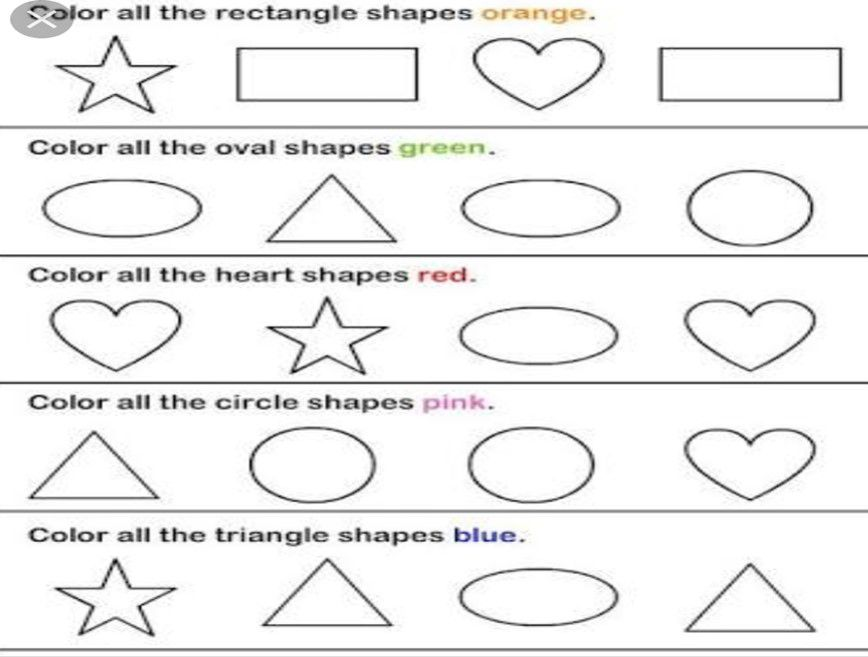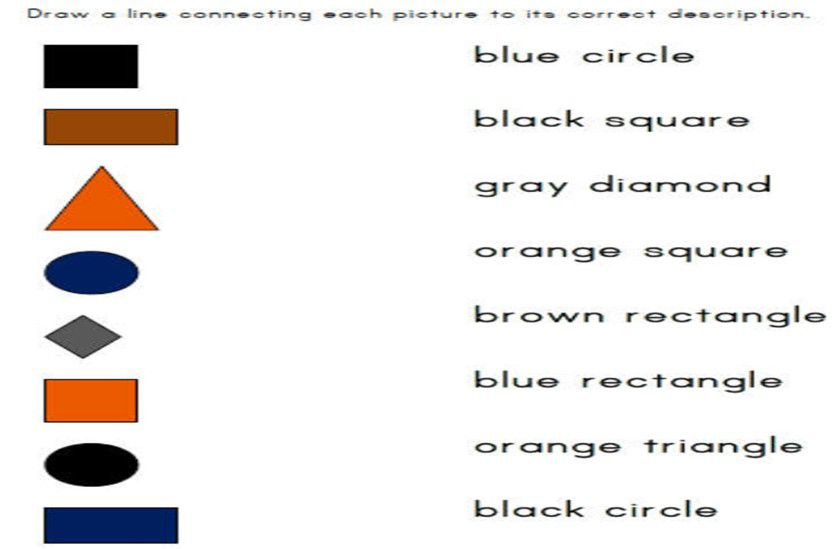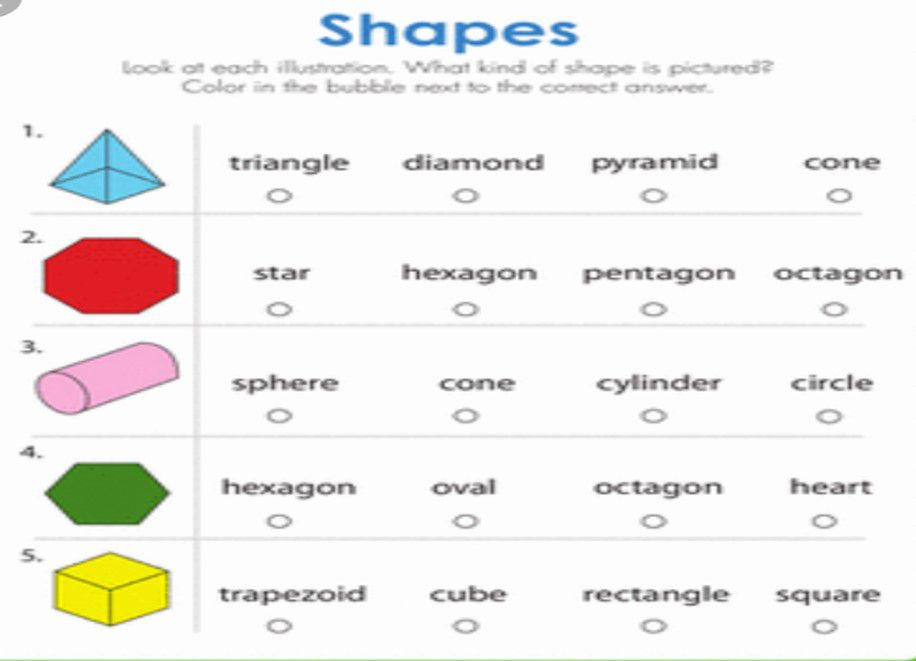Recap

• Geometrical skills is the ability of a person to determine shape of any figure.
• There are basic foue figures circle, square, triangle and rectangle.
• Circle is round in shape. Example – Apple, Orange, sun etc.
• Rectangle has four sides and it’s opposite sides are equal.
• Square has four sides all are equal.
• Traingle has three sides and is of shape of mountain.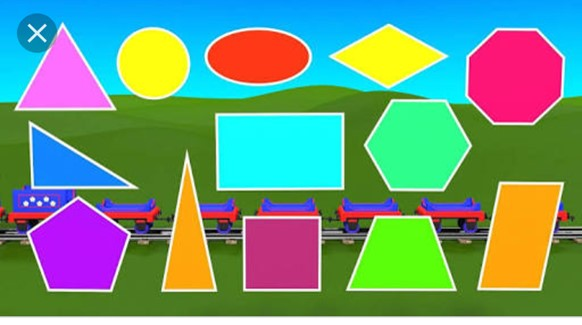## Quiz for Geometrical Skills

 Q.1 Identify the shape.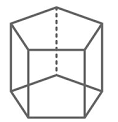a) Pentagonal prism b) Pentagonal pyramid c) Hexagonal prism d) Hexagonal pyramid
 Q.2 The basic shapes of the diagram are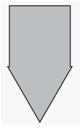a) Triangle b) Square c) Circle d) Both (a) and (b)
 Q.3 Which of the following is symmetric? a)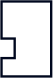b)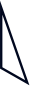c)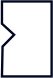d)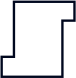Q.4 Which of the following letter is made of only straight lines? a)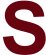b)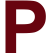c)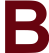d)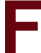Q.5 The diagram in the picture is a ________.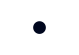a) Line b) Point c) Ray d) Line Segment
 Q.6 Count the number of triangles in the diagram.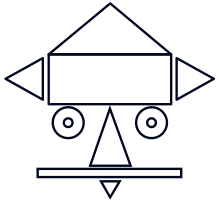a) 6 b) 5 c) 4 d) 3
 Q.7 Which of the following is a rectangular pyramid? a)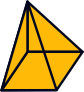b)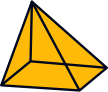c)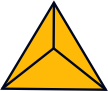d)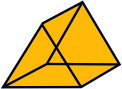Q.8 Identify the shape.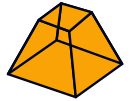a) Cone b) Prism c) Pyramid d) Frustum
 Q.9 If we fold the given paper along the lines, which geometrical shape will be obtained?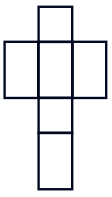a) Cube b) Rhombus c) Prism d) Cuboid
 Q.10 Count the number of squares in the given diagram.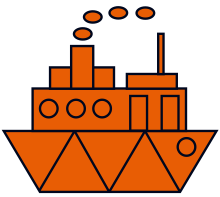a) 2 b) 3 c) 4 d) 5